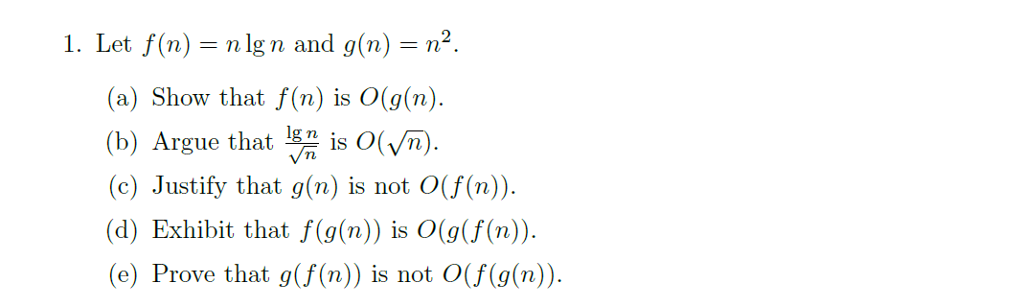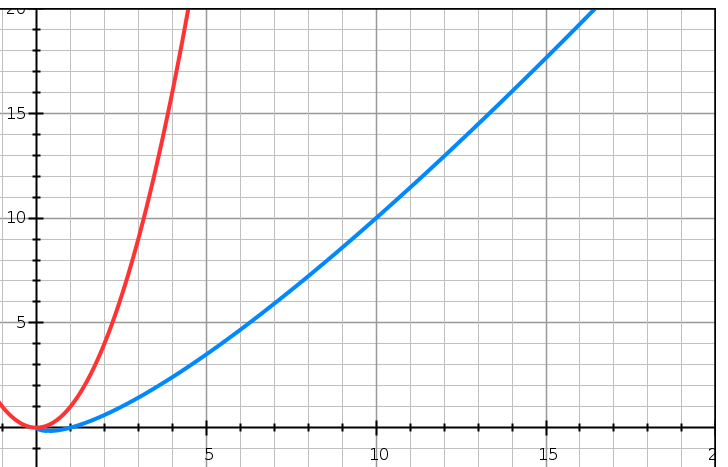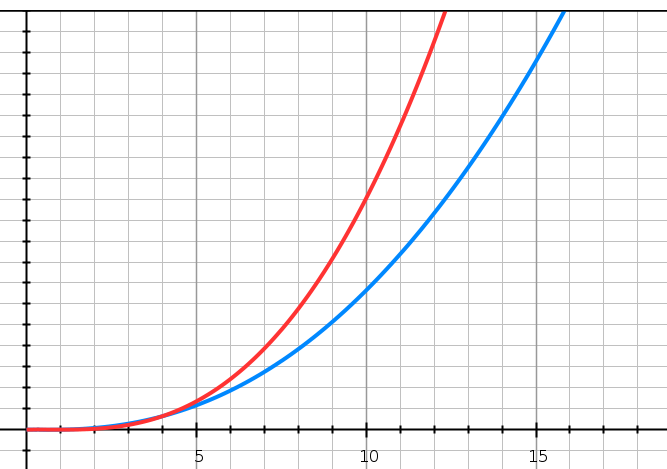# Question & Answer: Let f(n) = n lg n and g(n) = n^2. (a) Show that f(n) is O(g(n). (b) Argue that lg n/Squareroot n is O(…..Let f(n) = n lg n and g(n) = n^2. (a) Show that f(n) is O(g(n). (b) Argue that lg n/Squareroot n is O(Squareroot n). (c) Justify that g(n) is not O(f(n)). (d) Exhibit that f(g(n)) is O(g(f(n)). (e) Prove that g(f(n)) is not O(f(g(n)).

Don't use plagiarized sources. Get Your Custom Essay on
Question & Answer: Let f(n) = n lg n and g(n) = n^2. (a) Show that f(n) is O(g(n). (b) Argue that lg n/Squareroot n is O(…..
GET AN ESSAY WRITTEN FOR YOU FROM AS LOW AS \$13/PAGE

From defination, we know that Big-O notation denotes the upper bound or more formally a description of a function in terms of big O notation usually only provides an upper bound on the growth rate of the function.

1.
It is quite clear that nlogn grows less than n^2, so it is sensible saying
nlogn = O(n^2)
according to the defination provided abovered: n^2
blue: n*log(n)

2
Same goes here
We know that growth rate of (log n) < growthrate of (n)
similarlly growth rate of (log n/ sqrt(n)) < growthrate of (n/(sqrt(n))
or growth rate of (log n/ sqrt(n)) < growthrate of ((sqrt(n))

Simple mathematics! clear enough?

now according to defination we can say log n/sqrt(n) = O(sqrt(n))

so the stament is valid

3
As already said in question 1, growth rate of g(n) is more than growth rate of f(n) so g(n) is not O(f(n))

4.
f(g(n)) = n^2 log(n^2) = 2 n^2 log n
g(f(n)) = (n log n)^2 = n^2 log(n) * log(n)

So from the graph it is clear that growth rate of f(g(n)) < growth rate of g(f(n))

so it is valid that f(g(n)) > g(f(n))red: g(f(n)) = n * n * log(n) * log(n)
blue: f(g(n)) = 2 * n*n * log(n)

5.
Since 4 is valid, 5 is not valid, quite obhious!